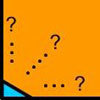Search by Topic

Filter by: Content type:
Age range:
Challenge level:

There are 10 NRICH Mathematical resources connected to Proof by contradiction, you may find related items under Using, Applying and Reasoning about Mathematics.

Broad Topics > Using, Applying and Reasoning about Mathematics > Proof by contradictionEyes Down

Age 16 to 18 Challenge Level:

The symbol [ ] means 'the integer part of'. Can the numbers [2x]; 2[x]; [x + 1/2] + [x - 1/2] ever be equal? Can they ever take three different values?Impossible Square?

Age 16 to 18 Challenge Level:

Can you make a square from these triangles?Rarity

Age 16 to 18 Challenge Level:

Show that it is rare for a ratio of ratios to be rational.An Introduction to Proof by Contradiction

Age 14 to 18

An introduction to proof by contradiction, a powerful method of mathematical proof.Rational Round

Age 16 to 18 Challenge Level:

Show that there are infinitely many rational points on the unit circle and no rational points on the circle x^2+y^2=3.Proof Sorter - the Square Root of 2 Is Irrational

Age 16 to 18 Challenge Level:

Try this interactivity to familiarise yourself with the proof that the square root of 2 is irrational. Sort the steps of the proof into the correct order.Staircase

Age 16 to 18 Challenge Level:

Solving the equation x^3 = 3 is easy but what about solving equations with a 'staircase' of powers?Tetra Inequalities

Age 16 to 18 Challenge Level:

Prove that in every tetrahedron there is a vertex such that the three edges meeting there have lengths which could be the sides of a triangle.Proximity

Age 14 to 16 Challenge Level:

We are given a regular icosahedron having three red vertices. Show that it has a vertex that has at least two red neighbours.Be Reasonable

Age 16 to 18 Challenge Level:

Prove that sqrt2, sqrt3 and sqrt5 cannot be terms of ANY arithmetic progression.